Get instant live expert help with Excel or Google Sheets“My Excelchat expert helped me in less than 20 minutes, saving me what would have been 5 hours of work!”

#### Post your problem and you'll get expert help in seconds

Your message must be at least 40 characters
Our professional experts are available now. Your privacy is guaranteed.

# Excel ROW Function

While working with Excel, we are able to determine the row number of any cell or reference by using the ROW function.  This step by step tutorial will assist all levels of Excel users in the usage and syntax of the ROW function.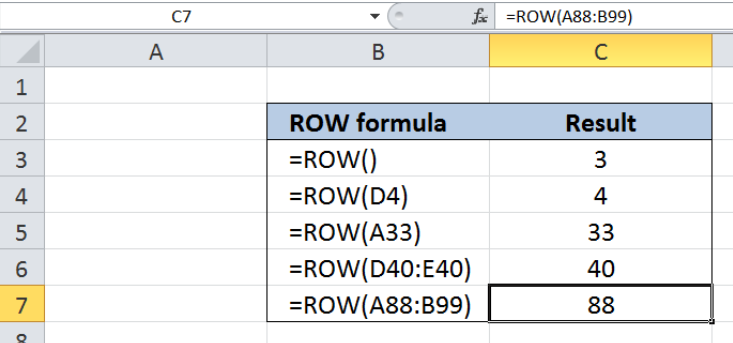Figure 1. Final result: Excel ROW function

## Syntax of the ROW function

ROW function returns the row number of a reference

`=ROW(reference)`

• reference – The cell or range of cells whose row number we want to determine
• When reference is not given, ROW function returns the row number of the cell containing the formula

## Setting up our Data

Our data consists of two columns: ROW formula (column B) and Result (column C).  We want to enter the ROW formulas in column B and see the results in column C.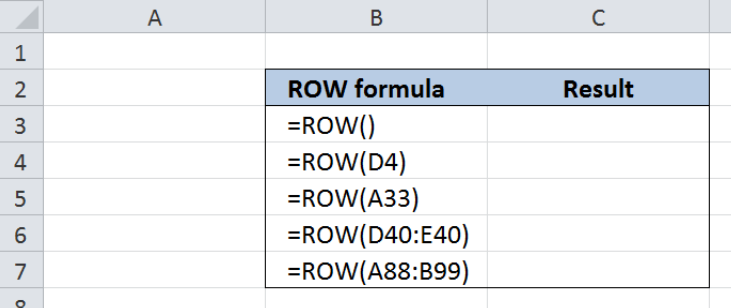Figure 2. Sample data to determine the row number

## Determine the row number of a certain cell

In order to determine the row number of a certain cell containing the ROW function, we leave the reference argument blank. Let us follow these steps:

Step 1.  Select cell C3

Step 2.  Enter the formula: `=ROW()`

Step 3.  Press ENTER

As a result, the value in cell C3 is 3, because cell C3 is in the third row of the worksheet.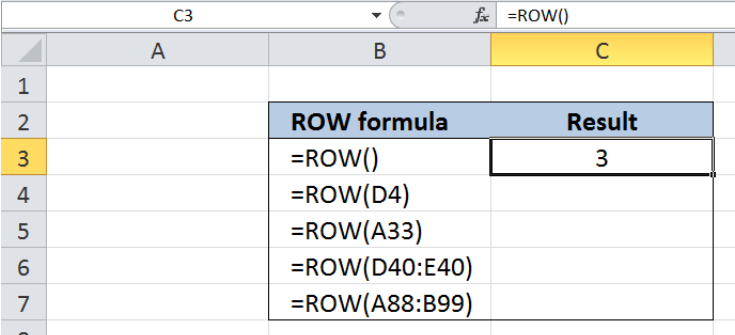Figure 3.  Using ROW function to determine the row number of a certain cell

## Determine the row number of any cell

In order to determine the row number of any cell in the worksheet,  we use the ROW function and enter the cell reference as the argument.  We want to know the row number of cell D4. Let us follow these steps:

Step 1.  Select cell C4

Step 2.  Enter the formula: `=ROW(D4)`

Step 3.  Press ENTER

As a result, the value in C4 is 4, because cell D4 is in the fourth row of the worksheet.  ROW function returns only the row number, regardless of the column number of the cell.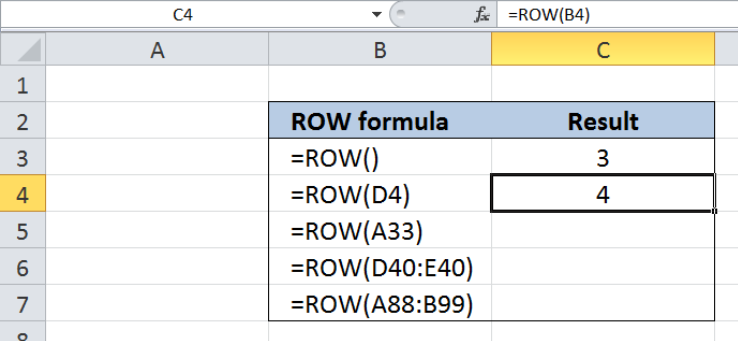Figure 4.  Using ROW function to determine the row number of D4

In a similar manner, entering the formula =ROW(A33) in cell C5 will result to the value “33”, because A33 is located in the 33rd row of the worksheet.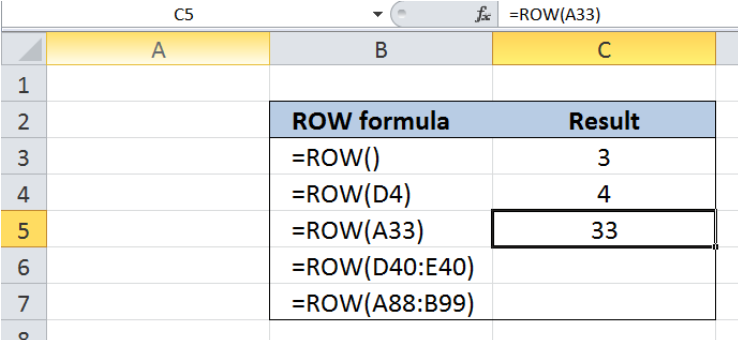Figure 5.  Using ROW function to determine the row number of A33

## Determine the row number with range as reference

When a range is used as a reference argument in the ROW function, the value returned is the row number of the upper leftmost cell in the range.  Let us follow these steps:

Step 1.  Select cell C6

Step 2.  Enter the formula: `=ROW(D40:E40)`

Step 3.  Select cell C7

Step 4.  Enter the formula: `=ROW(A88:B99)`

Step 5.  Press ENTER

As a result, the value in cell C6 is 40, because cell D40 is the upper leftmost cell in the range D40:E40 and its row number is 40.  In cell C7, the resulting value is 88, because A88 is the upper leftmost cell in the range A88:B99 and its row number is 88.

Below table shows the results in cells C3:C7, where the row number of the given cells is returned by the ROW function.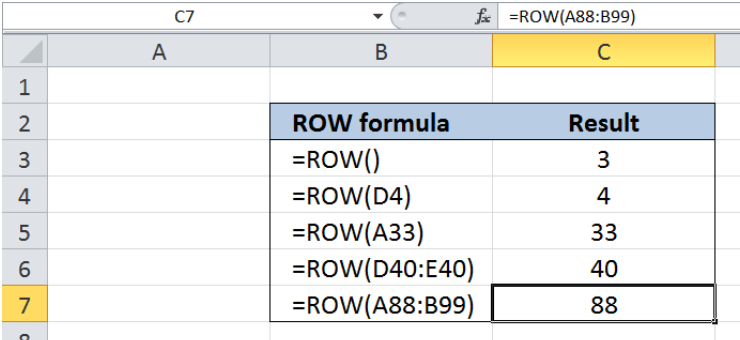Figure 6.  Output: Row number with range as reference argument

## Instant Connection to an Expert through our Excelchat Service

Most of the time, the problem you will need to solve will be more complex than a simple application of a formula or function. If you want to save hours of research and frustration, try our live Excelchat service! Our Excel Experts are available 24/7 to answer any Excel question you may have. We guarantee a connection within 30 seconds and a customized solution within 20 minutes.

### Did this post not answer your question? Get a solution from connecting with the expert.Another blog reader asked this question today on Excelchat:## Subscribe to Excelchat.coAnother blog reader asked this question today on Excelchat: